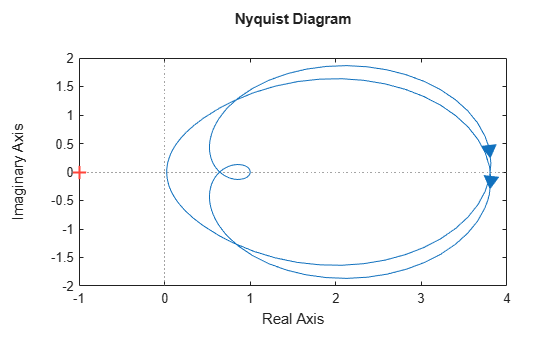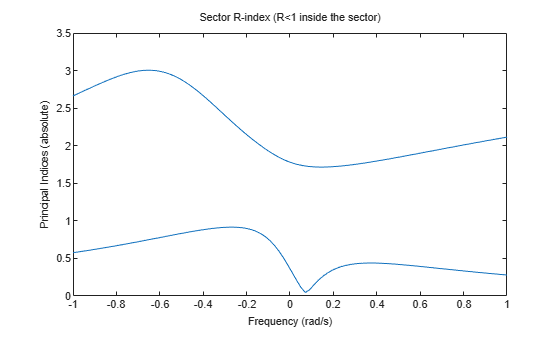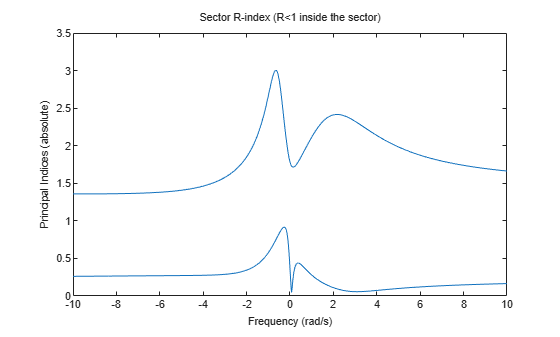# getPassiveIndex

Compute passivity index of linear system

## Syntax

``R = getPassiveIndex(G)``
``nu = getPassiveIndex(G,'input')``
``rho = getPassiveIndex(G,'output')``
``tau = getPassiveIndex(G,'io')``
``DX = getPassiveIndex(G,dQ)``
``index = getPassiveIndex(___,tol)``
``index = getPassiveIndex(___,tol,fband)``
``[index,FI] = getPassiveIndex(___)``
``````[index,FI,Qout,dQout] = getPassiveIndex(___)``````

## Description

`getPassiveIndex` computes various measures of the excess or shortage of passivity for a given system.

A linear system G(s) is passive if all its I/O trajectories (u(t),y(t)) satisfy:

`${\int }_{0}^{T}y{\left(t\right)}^{T}u\left(t\right)dt>0,$`

for all T > 0. Equivalently, a system is passive if its frequency response is positive real, such that for all ω > 0,

`$G\left(j\omega \right)+G{\left(j\omega \right)}^{H}>0$`

(or the discrete-time equivalent).

example

````R = getPassiveIndex(G)` computes the relative passivity index. `G` is passive when `R` is less than one. `R` measures the relative excess (`R` < 1) or shortage (`R` > 1) of passivity.For more information about the notion of passivity indices, see About Passivity and Passivity Indices.```

example

````nu = getPassiveIndex(G,'input')` computes the input passivity index. The system is input strictly passive when `nu` > 0. `nu` is also called the input feedforward passivity (IFP) index. The value of `nu` is the minimum feedforward action such that the resulting system is passive.For more information about the notion of passivity indices, see About Passivity and Passivity Indices.```

example

````rho = getPassiveIndex(G,'output')` computes the output passivity index. The system is output strictly passive when `rho` > 0. `rho` is also called the output feedback passivity (OFP) index. The value of `rho` is the minimum feedback action such that the resulting system is passive.For more information about the notion of passivity indices, see About Passivity and Passivity Indices.```

example

````tau = getPassiveIndex(G,'io')` computes the combined I/O passivity index. The system is very strictly passive when `tau` > 0.For more information about the notion of passivity indices, see About Passivity and Passivity Indices.```
````DX = getPassiveIndex(G,dQ)` computes the directional passivity index in the direction specified by the matrix `dQ`.```
````index = getPassiveIndex(___,tol)` computes the passivity index with relative accuracy specified by `tol`. Use this syntax with any of the previous combinations of input arguments. `index` is the corresponding passivity index `R`, `nu`, `rho`, `tau`, or `DX`.```
````index = getPassiveIndex(___,tol,fband)` computes passivity indices restricted to a specified frequency interval.```

example

````[index,FI] = getPassiveIndex(___)` also returns the frequency at which the returned index value is achieved.```
``````[index,FI,Qout,dQout] = getPassiveIndex(___)``` also returns the sector matrix `Qout` for passivity and the directional index matrix `dQout`.```

## Examples

collapse all

Compute passivity indices for the following dynamic system:

`$G\left(s\right)=\frac{{s}^{2}+s+5s+0.1}{{s}^{3}+2{s}^{2}+3s+4}$`

`G = tf([1,1,5,.1],[1,2,3,4]);`

Compute the relative passivity index.

`R = getPassiveIndex(G)`
```R = 0.9512 ```

The system is passive, but with a relatively small excess of passivity.

Compute the input and output passivity indices.

`nu = getPassiveIndex(G,'input')`
```nu = 0.0250 ```
`rho = getPassiveIndex(G,'output')`
```rho = 0.2583 ```

This system is both input strictly passive and output strictly passive.

Compute the combined I/O passivity index.

`tau = getPassiveIndex(G,'io')`
```tau = 0.0250 ```

The system is very strictly passive as well. A system that is very strictly passive is also strictly positive real. Examining the Nyquist plot confirms this, showing that the frequency response lies entirely within the right half-plane.

`nyquistplot(G)`The relatively small `tau` value is reflected in how close the frequency response comes to the imaginary axis.

For systems with complex coefficients, `getPassiveIndex` can return indices at a negative or positive frequency depending on the `fband` you specify.

Load the state-space model with complex data.

`load compCoeffModel.mat`

Compute the relative passivity index and its frequency with a relative accuracy of 0.0001%. Also, specify `fband` = `[0.1,1]` to compute the index in the frequency interval [–1,–0.1] ∪ [0.1,1].

`[R,FI] = getPassiveIndex(sys,1e-6,[0.1,1])`
```R = 3.0050 ```
```FI = -0.6518 ```

In this interval, `sys` achieves a relative passivity index of 3.0050 at a negative frequency value of –0.6518 rad/s. Use `passiveplot` to plot the indices in this range.

```opt = sectorplotoptions; opt.FreqScale = 'Linear'; opt.IndexScale = 'Linear'; w = linspace(-1,1,100); passiveplot(sys,w,opt)```Now compute the relative passivity index in the frequency interval [–10,–1.5] ∪ [1.5,10]. To do so, specify `fband` = `[1.5,10]`.

`[R,FI] = getPassiveIndex(sys,1e-6,[1.5,10])`
```R = 2.4162 ```
```FI = 2.1707 ```

In this interval, `sys` achieves a relative passivity index of 2.4162 at a positive frequency value of 2.1707 rad/s. Plot the indices in this range to confirm the result.

```w = linspace(-10,10,1000); passiveplot(sys,w,opt)```## Input Arguments

collapse all

Model to analyze for passivity, specified as a dynamic system model such as a `tf`, `ss`, or `genss` model. `G` can be MIMO, if the number of inputs equals the number of outputs. `G` can be continuous or discrete. If `G` is a generalized model with tunable or uncertain blocks, `getPassiveIndex` evaluates passivity of the current, nominal value of `G`.

If `G` is a model array, then `getPassiveIndex` returns the passivity index as an array of the same size, where:

`index(k) = getPassivityIndex(G(:,:,k),___)`

Here, `index` is any of `R`, `nu`, `rho`, `tau`, or `DX`, depending on which input arguments you use.

Custom direction in which to compute passivity, specified as a symmetric square matrix that is `2*ny` on a side, where `ny` is the number of outputs of `G`.

The `rho`, `nu`, and `tau` indices each correspond to a particular direction in the y/u space of the system, with a corresponding `dQ` value. (See `dQout` for these values.) Use this argument to specify your own value for this direction.

Relative accuracy for the calculated passivity index. By default, the tolerance is 1%, meaning that the returned passivity index is within 1% of the actual passivity index.

Frequency interval for determining passivity index, specified as an array of the form `[fmin,fmax]` with 0 ≤ `fmin` < `fmax`. When you provide `fband`, then `getPassiveIndex` restricts the frequency-domain computation of the passivity index to that frequency range. For example, the relative passivity index `R` is the peak gain of the bilinear-transformed system `(I-G)(I+G)^-1` (for minimum-phase `(I + G)`). When you provide `fband`, then `R` is the peak gain within the frequency band.

For models with complex coefficients, `getPassiveIndex` computes the passivity index in the range `[–fmax,–fmin]``[fmin,fmax]`. As a result, the function can return indices at a negative frequency.

Specify frequencies in units of `rad/TimeUnit`, where `TimeUnit` is the `TimeUnit` property of the dynamic system model `G`.

## Output Arguments

collapse all

Relative passivity index, returned as a scalar, or an array if `G` is an array.

The system `G` is passive when `R` is less than one.

• `R` < 1 indicates a relative excess of passivity.

• `R` > 1 indicates a relative shortage of passivity.

When `I + G` is minimum phase, `R` is the peak gain of the bilinear-transformed system ```(I - G)(I + G)^-1```.

Input passivity index, returned as a scalar, or an array if `G` is an array. `nu` is defined as the largest value of ν for which:

`${\int }_{0}^{T}y{\left(t\right)}^{T}u\left(t\right)dt>\nu {\int }_{0}^{T}u{\left(t\right)}^{T}u\left(t\right)dt,$`

for all T > 0. Equivalently, `nu` is the largest ν for which:

`$G\left(j\omega \right)+G{\left(j\omega \right)}^{H}>2\nu I$`

(or the discrete-time equivalent). The system is input strictly passive when `nu` > 0. `nu` is also called the input feedforward passivity (IFP) index. The value of `nu` is the minimum feedforward action such that the resulting system is passive.

Output passivity index, returned as a scalar, or an array if `G` is an array. `rho` is defined as the largest value of ρ for which:

`${\int }_{0}^{T}y{\left(t\right)}^{T}u\left(t\right)dt>\rho {\int }_{0}^{T}y{\left(t\right)}^{T}y\left(t\right)dt,$`

for all T > 0. The system is output strictly passive when `rho` > 0. `rho` is also called the output feedback passivity (OFP) index. The value of `rho` is the minimum feedback action such that the resulting system is passive.

Combined I/O passivity index, returned as a scalar, or an array if `G` is an array. `tau` is defined as the largest value of τ for which:

`${\int }_{0}^{T}y{\left(t\right)}^{T}u\left(t\right)dt>\tau {\int }_{0}^{T}\left(u{\left(t\right)}^{T}u\left(t\right)+y{\left(t\right)}^{T}y\left(t\right)\right)dt,$`

for all T > 0. The system is very strictly passive when `tau` > 0.

Directional passivity index in the direction specified by `dQ`, returned as a scalar, or an array if `G` is an array. The directional passivity index is the largest value of D for which:

`${\int }_{0}^{T}y{\left(t\right)}^{T}u\left(t\right)dt>D{\int }_{0}^{T}\left({\left(\begin{array}{c}y\left(t\right)\\ u\left(t\right)\end{array}\right)}^{T}dQ\left(\begin{array}{c}y\left(t\right)\\ u\left(t\right)\end{array}\right)\right)dt,$`

for all T > 0. The `rho`, `nu`, and `tau` indices correspond to particular choices of dQ (see the output argument `dQout`). To compute `DX`, the software uses the custom dQ value you supply, `dQ`.

Frequency at which the returned passivity index is achieved, returned as a scalar, or an array if `G` is an array. In general, the passivity indices vary with frequency (see `passiveplot`). For each index type, the returned value is the largest value over all frequencies. `FI` is the frequency at which this value occurs, returned in units of `rad/TimeUnit`, where `TimeUnit` is the `TimeUnit` property of `G`.

`FI` can be negative for systems with complex coefficients.

Sector geometry used for computing the passivity index, returned as a matrix. For passivity indices, `Qout` is given by:

`Qout = [zeros(ny),-1/2*eye(ny);-1/2*eye(ny),zeros(ny)]; `

where `ny` is the number of outputs of `G`. For example, for a SISO `G`,

```Qout = [ 0, -0.5; -0.5, 0 ];```

For more information about sector geometry, see `getSectorIndex`.

Direction in which passivity is computed, returned as a square matrix that is `2*ny` on a side, where `ny` is the number of outputs of `G`. The value returned for `dQout` depends on what kind of passivity index you calculate:

• `nu` — For the input passivity index, `dQout` is given by:

`dQout = [zeros(ny),zeros(ny);zeros(ny),eye(ny)];`

For instance, for a SISO system, ```dQout = [0,0;0,1]```.

• `rho` — For the output passivity index, `dQout` is given by:

`dQout = [eye(ny),zeros(ny);zeros(ny),zeros(ny)];`

For instance, for a SISO system, ```dQout = [1,0;0,0]```.

• `tau` — For the combined I/O passivity index, `dQout` is given by:

`dQout = eye(2*ny);`

For instance, for a SISO system, ```dQout = [1,0;0,1]```.

• `DX``dQout` is the custom value you provide in the `dQ` input argument.

• `R` — The relative passivity index does not involve a direction, so in this case the function returns `dQout = []`.

For more information about directional indices, see `getSectorIndex`.

## Limitations

• `getPassiveIndex(G,'output')` might return incorrect results when one of the following conditions occurs:

• `G^-1` is improper, that is, has infinite gain at `s = Inf` or ```z = Inf```.

• `G^-1` has poles on the imaginary axis (for continuous-time `G`) or on the unit circle (for discrete-time `G`).

To circumvent this limitation, perturb `G` to have as many zeros as poles and only stable zeros. For example:

```G = tf([1 0],[1 0.2 1]); [rho,freq] = getPassiveIndex(G,'output') % returns incorrect answer zpk(G+1e-4) % perturbed G is bi-proper and has stable zeros [rho,freq] = getPassiveIndex(G+1e-4,'output') % returns expected answer ```

 Xia, M., P. Gahinet, N. Abroug, C. Buhr, and E. Laroche. “Sector Bounds in Stability Analysis and Control Design.” International Journal of Robust and Nonlinear Control 30, no. 18 (December 2020): 7857–82. https://doi.org/10.1002/rnc.5236.